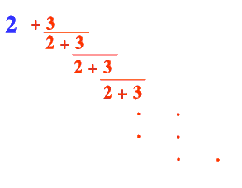#### You may also like### Good Approximations

Solve quadratic equations and use continued fractions to find rational approximations to irrational numbers.### Not Continued Fractions

Which rational numbers cannot be written in the form x + 1/(y + 1/z) where x, y and z are integers?### Comparing Continued Fractions

Which of these continued fractions is bigger and why?

# There's a Limit

##### Age 14 to 18Challenge Level

Tony of State College Area High School, Pennsylvania, USA and Chi Kin of Saint Dominic's International School, Lisbon sent in excellent solutions and here is Chi Kin's:

What happens when successive terms are taken in the continued fractionI calculated the first five terms

$2 + {3\over2} = {7\over2} = 3.5$

$2 + \frac{3}{2+{3\over2}} = {20\over7} = 2.86$

The third term is $3.05$

The fourth term is $2.95$

And the fifth term is $3.02$

Thus, when successive terms are taken, the results oscillate alternately above and below $3$.

Indeed, if the fraction goes on forever, we would get $3$.

Suppose the continued fraction is denoted as $F$. Thus

\eqalign{ F &=& 2 + \frac{3}{F} \\ F &=& \frac{2F + 3}{F} \\ F^2 - 2F - 3 &=& 0 \\ (F-3)(F+1) &=& 0.}

Thus $F = 3$, because $F$ cannot be negative.